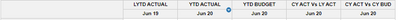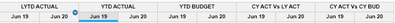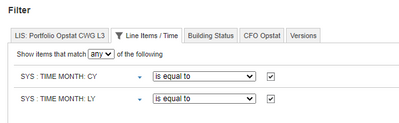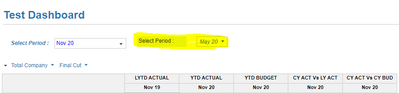# Period shown module automatically update when i update the time selectorHello,

Is there a way I can automatically change the shown period in my module when I change the time selected?

See screenshot attached. I need to show the period in each line item.Thanks,

Tom

Tagged:

•Ok.

Then I would create a line item subset (LIS) and use this in your filter as an additional dimension.

Use a IF THEN function to determine which formula is allocated to each line item.

= IF ITEM(LIS) = LYTD ACTUAL THEN ITEM(TIME) = PERIOD(CURRENTPERIODEND()) - 12 ELSE ITEM(TIME) = PERIOD(CURRENTPERIODEND())

You will only need one line item using the above

•You can use a time system module to create a filter.

I will assume that the time period is the current period as set in the model time settings.

Therefore, create a module dimensioned only by time period month.

Create a line item called 'Current Period' and format this as a boolean.

Use the following formula = ITEM(TIME) = PERIOD(CURRENTPERIODEND())

This will check the current period as per the model time settings.

You can now use this to filter your time dimension in the target module.

Edit - I notice that you also need last year.

Create a second line item called, 'Last year.'

Use the following formula = ITEM(TIME) = PERIOD(CURRENTPERIODEND()) - 12

Create a third line item called, 'Final Filter.'

Use the following formula = Current Period OR Last Year

Use this, 'Final Filter,' in your target module.

PS. I would swap your line items and time in the pivot so that time sits above. It will present better in the resulting table.

•Hi @ChrisAHeathcote , i have done that but i still need to manually hide the other period in the module.

See below screenshots.Thanks for helping.

Tom

•Hi @ChrisAHeathcote , thanks that solution works.

In addition, is there a function I can use in the Time system module that will auto-update based on the time selector in the dashboard?Regards,

Tom

•To synch the filter to the time selection you need to base the function on that line item.

From the screen shot I assume you have published a line item format to time period month.

Instead of referencing PERIOD(CURRENTPERIODEND()) just reference that line item.

When you select from the drop down the filter should automatically update.